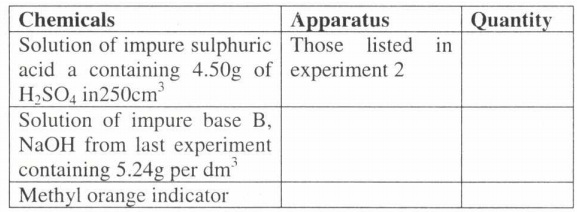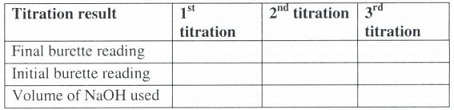#### Determination of percent purity of substance, Chemistry tutorial

Introduction:

The previous experiments in acid - base titrations had exposed us to the laboratory skills required to successfully titrate an acid against a base. We have also learnt how to calculate the volume and molar concentrations of either of the reactants. In particular we are fully aware of the standardization procedure required to know the actual concentration of a solution whose concentration is not accurately known.

However, acid - base titrations provide more applications than this. We can also use titrimetric analysis to determine other parameters such as the percentage purity of a substance. Sometimes, one of the substances used in the titrations may be impure. This impurity may be due to improper preparations of the substance e.g. presence of water of crystallization or contamination by one of its salts e.g. contamination of NaOH via NaCl.

In any of these cases however, the neutralization reaction will only occur between the pure component of the impure substance and the pure titrant. That is, the impurity will remain in solution. In the specific cases mentioned above, the contaminant, NaCl and the water of crystallization will remain in solution whilst the pure NaOH will react with the titrant.

Experiment:

Determination of the percentage purity of a substance

Requirements:Procedure:

1. Rinse a burette twice through a few drops of solution A and next fill it by similar solution above the zero mark. Drain to the marking, making sure the burette is full.

2. Using a pipette, move 20cm3 of solution B into a conical flask. Add 2 to 3 drops of methyl orange. Place the conical flask into a white sheet of paper or tile.

3. Run solution A to the conical flask whilst shaking vigorously until a permanent faint pink coloration is examined.

4. Replicate the titration using fresh portions of solution B until at least 2 titrations are within the accuracy of 2.2cm 3 of the titrant.

5. Discover the average of the titre values.

Problem:

From your average titre value, determine

a) The molar concentration of solution B

b) The amount in moles of solution A

c) The molar concentration of solution A

d) The mass concentration of the pure acid in solution A in grams per dm3

e) The percentage impurity of the solution A

f) Name another indicator that might be suitable for this reaction and what would be the colour at the end point.

(Na = 23, 0 = 16, H = 1, S =32)

Results:Average titre reading = + + /3 = 22.50cm3   (assuming)

Treatment of Results:

This is the 1st in a series of actual practical test. The workings have been provided to enable us additional understand the steps involved in the calculation. Note that: the titre value 1 have used might be different from the titre value we actually gain.

The balanced chemical equation is

2NaOH + H2SO4     →    Na2O4   + 2H2O

The mass concentration of solution B = 5.24g dm-3

Molar mass of NaOH = 40.0g.mol -1

a) Molar conc. of solution B =  mass/ molar mass  =  5.24 gdm-3  / 40g mo1 -1

= 0.131.moldm-3

b) The amount in moles of solution A.

First, we find the amount in moles of B = molar conc. X volume

= 0.131 moldm-3   x 20dm3/1000

= 0.0026 mole

From stiochiometry

2 moles of B = 1 mole of A

•.0.0026 moles of B = 1/2  (0.0026 moles of A) / 0.0013 moles of A

c) The molar concentration of A

= 0.0013 moles of A is contained in say, 22.50cm3

1000cm 3 will contain 0.0013   x 1000 /22.50

= 0.058 moles dm-3

d) The mass concentration of the pure acid in grams per dm3 molar mass H2SO4 = 2 + 32 + 64 = 98.0 per mole mass concentration = 0.0058 x 98 = 5.706gdm-3

e) The percentage impurity of solution A we must find the concentration of the original impure acid in g/dm3, We were provided with 4.50g in 250cm3 250cm3 of solution A contains 4.50g 1000cm3   dm3) will contain 4.50 x 1000gdm-3 /250

The concentration in gdm-3     =18.0gdm3

But the mass of solution A obtained from the titration is 5.706gdm

Mass of the impurity  = 18.0 - 5.7

= 1/.3gclm-3

The percent impurity = mass of impurity x 100 mass of impure acid

= 12.3 x100 /18.0

= 68.33%

That is, solution A is 68.33% impure.

f) This question was asked to enable you choose indicators in unit 5 and to make you aware that there could be other suitable indicators. Another suitable indicator is phenolphthalein indicator and the colour at the end point is colourless.

Tutorsglobe: A way to secure high grade in your curriculum (Online Tutoring)